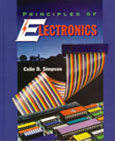# Principles of Electronics TextbookTextbook to Accompany Electronics Technician CBT
Copyright 2011, 760 pg
Hardcover
ISBN 0-9686860-0-1

Written by Colin Simpson, award-winning author of five textbooks in the electronics field, the book Principles of Electronics is designed to accompany the Electronics Technician CBT and offers a concise and practical overview of the basic principles, theorems, circuit behavior and problem-solving procedures of Electronic circuits and devices. The textbook reinforces concepts with practical "real-world" applications as well as mathematical solution, allowing readers to more easily relate the academic to the actual.

Assuming that readers have a basic understanding of algebra and trigonometry, Simpson offers a concise and practical overview of the basic principles, theorems, circuit behavior and problem-solving procedures. In one volume, this carefully developed text takes students from basic electricity through dc/ac circuits, semiconductors, operational amplifiers, and digital circuits. The book contains relevant, up-to-date information, giving students the knowledge and problem-solving skills needed to successfully obtain employment in the electronics field.

In addition, the book smoothly integrates the flow of material in a primarily non-mathematical style and includes features such as:

• Learning objectives that specify the chapters goals.
• Section reviews with answers at the end of each chapter.
• A comprehensive glossary.
• Hundreds of examples and end-of-chapter problems that illustrate fundamental concepts.
• Detailed chapter summaries.
• Practical Applications section which opens each chapter, presenting real-world problems and solutions.

Buy Now »